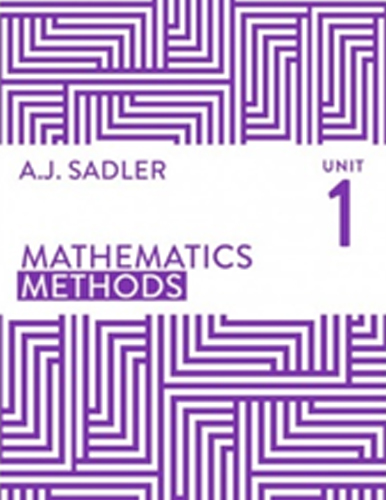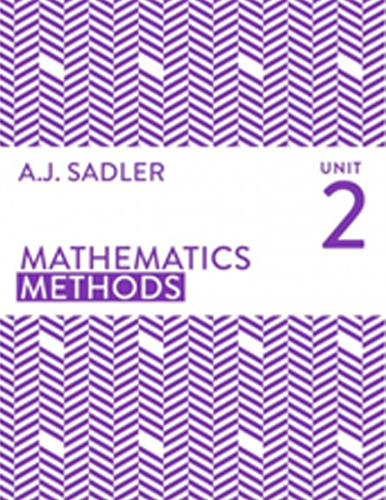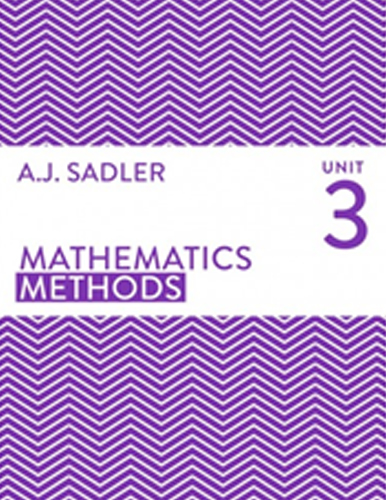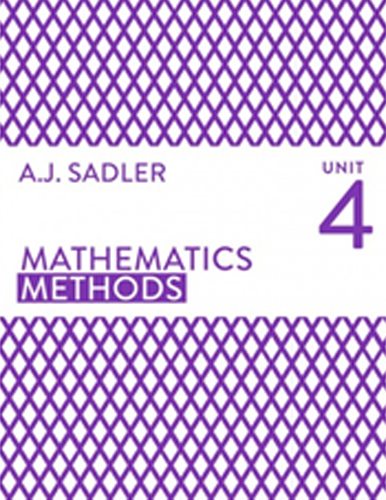## Mathematics Methods

Home / Mathematics Methods

# Mathematics Methods

## Mathematics Methods (ATAR) Tutor Perth

At Tutor Perth we hand pick the finest Maths Methods home tutors in Perth. Our Maths Methods tutors are engaging role models, mentors and are genuine specialists at teaching maths. We teach in accordance to the WA curriculum framework and provide comprehensive tutoring solutions to the A.J Sadler suite of text books, units 1, 2, 3 and 4.

Our engaging maths tutors help students achieve top ATAR results in the following A.J Sadler units:

## Year 11 Mathematics Methods (ATAR) | Specialist Tutors

### Tutoring – Unit 1

• Trigonometry
• Functions
• Linear functions
• Polynomials and other functions
• Trigometric functions
• Sets and Probability
• Counting### Tutoring – Unit 2

• Indices
• Exponential functions
• Sequences
• Series
• Rates of change
• Applications of differentiation
• Anti-differentiation
• Rectilinear motion

## Year 12 Mathematics Methods (ATAR) | Specialist Tutors### Tutoring – Unit 3

• Differentiation
• Applications of differentiation
• Anti-differentiation
• Area under a curve
• The fundamental theorem of calculus
• The exponential function
• Calculus of trigonometric functions
• Discrete random variables
• Bernoulli and Binomial distributions

### Tutoring – Unit 4

• Logarithmic functions
• Calculus involving logarithmic functions
• Continuous random variables
• The normal distribution
• Random sampling
• Sample proportions